SUM AND DIFFERENCE IDENTITIES
DOUBLE ANGLE AND HALF ANGLE IDENTITIESLearning Objectives - This is what you must know after studying the lecture and doing the practice problems!

1.   Given the formulas for the Sum Identity, be able to work with them.
2.   Given the formulas for the Difference Identity, be able to work with them.
3.   Given the formulas for the Double Angle Identity, be able to work with them.
4.   Given the formulas for the Half Angle Identity, be able to work with them.So far we were exposed several useful trigonometric identities. Namely, the Reciprocal, Quotient, and Pythagorean Identities. We will now discuss a few other identities that can also be useful at times.

They DO NOT have to be memorized! However, you must know how to work with them when given to you.

Proofs are provided separately for some of the formulas for your information only. If you are interested you might want to look at them. They can be found under the link "Point of Interest 6" in the Learning Materials #14 in the MyOpenMath course.

Sum Identities - see #1 through 3 in the "Examples" document

Let u and v be any algebraic expression.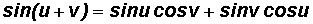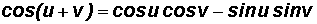Difference Identities - see #1 through 3 in the "Examples" document

Let u and v be any algebraic expression.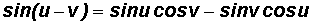Double Angle Identity for Sine - see #4 and 9 in the "Examples" document

Let u be any algebraic expression.Double Angle Identity for Cosine - see #5 and 6 in the "Examples" document

Let u be any algebraic expression.

Primary Identity: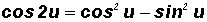Secondary Identities (derived from manipulating the primary identity):

1. Replace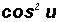in the primary identity with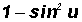(from Pythagorean Identity)

then2. Replace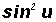in the primary identity with(from Pythagorean Identity)

thenDouble Angle Identity for Tangent:

There are other ways to show the Double Angle Identity for tangent, however, this is the easiest one and also the one we will use!

Let u be any algebraic expression.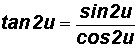Half-Angle Identity for Sine - see #7 and 9 in the "Examples" document

Let u be any algebraic expression.

Primary Identity:Secondary Identities (derived from manipulating the primary identity):

1. Replacein the primary identity with(from Pythagorean Identity)

then2. Replacein the primary identity with(from Pythagorean Identity)

thenLet u be any algebraic expression.Half-Angle Identity for Cosine - see #8 in the "Examples" document

Let u be any algebraic expression.

Let u be any algebraic expression.Half-Angle Identity for Tangent:

There are other ways to show the Half Angle Identity for tangent, however, this is the easiest one and also the one we will use!

Let u be any algebraic expression.NOTE: The plus or minus sign in the half angle identities depends on the quadrant in which the angle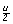lies.